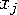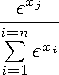Functions and CALL Routines

# CALL SOFTMAX Routine

Returns the softmax value.
 Category: Mathematical

## Syntax

 CALL SOFTMAX(argument<,argument,...>);

### Arguments

argument

is numeric.

 Restriction: The CALL SOFTMAX routine only accepts variables as valid arguments. Do not use a constant or a SAS expression because the CALL routine is unable to update these arguments.

The CALL SOFTMAX routine replaces each argument with the softmax value of that argument. For exampleis replaced byIf any argument contains a missing value, then CALL SOFTMAX returns missing values for all the arguments. Upon a successful return, the sum of all the values is equal to 1.

The following SAS statements produce these results:

SAS Statements Results
```x=0.5;
y=-0.5;
z=1;
call softmax(x,y,z);
put x= y= z=;```
```

x=0.3314989604 y=0.1219516523 z=0.5465493873```Previous Page | Next Page | Top of Page